# Pythagorean theorem + analytic geometry - math problems

#### Number of problems found: 62

• Hyperbola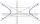Find the equation of hyperbola that passes through the point M [30; 24] and has focal points at F1 [0; 4 sqrt 6], F2 [0; -4 sqrt 6].
• Three pointsThree points A (-3;-5) B (9;-10) and C (2;k) . AB=AC What is value of k?
• Calculate 6Calculate the distance of a point A[0, 2] from a line passing through points B[9, 5] and C[1, -1].
• Unit vector 2DDetermine coordinates of unit vector to vector AB if A[-6; 8], B[-18; 10].
• On lineOn line p: x = 4 + t, y = 3 + 2t, t is R, find point C, which has the same distance from points A [1,2] and B [-1,0].
• Parametric formCalculate the distance of point A [2,1] from the line p: X = -1 + 3 t Y = 5-4 t Line p has a parametric form of the line equation. ..
• Calculate 7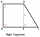Calculate the height of the trapezoid ABCD, where coordinates of vertices are: A[2, 1], B[8, 5], C[5, 5] and D[2, 3]
• On a lineOn a line p : 3 x - 4 y - 3 = 0, determine the point C equidistant from points A[4, 4] and B[7, 1].
• Perpendicular projectionDetermine the distance of a point B[1, -3] from the perpendicular projection of a point A[3, -2] on a straight line 2 x + y + 1 = 0.
• Equation of circlefind an equation of the circle with indicated properties: a. center at (-3,5), diameter 20. b. center at origin and diameter 16.
• Vertices of a right triangleShow that the points D(2,1), E(4,0), F(5,7) are vertices of a right triangle.
• Diameter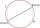If the endpoints of a diameter of a circle are A(10, -1) and B (3, 10), what is the radius of the circle?
• Touch x-axisFind the equations of circles that pass through points A (-2; 4) and B (0; 2) and touch the x-axis.
• Center of line segmentCalculate the distance of the point X [1,3] from the center of the line segment x = 2-6t, y = 1-4t ; t is .
• Chord BC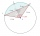A circle k has the center at the point S = [0; 0]. Point A = [40; 30] lies on the circle k. How long is the chord BC if the center P of this chord has the coordinates: [- 14; 0]?
• Inscribed circle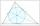Write the equation of a incircle of the triangle KLM if K [2,1], L [6,4], M [6,1].
• 3d vector componentThe vector u = (3.9, u3) and the length of the vector u is 12. What is is u3?
• Isosceles triangleIn an isosceles triangle ABC with base AB; A [3,4]; B [1,6] and the vertex C lies on the line 5x - 6y - 16 = 0. Calculate the coordinates of vertex C.
• Equation of circle 2Find the equation of a circle which touches the axis of y at a distance 4 from the origin and cuts off an intercept of length 6 on the axis x.
• Medians and sidesTriangle ABC in the plane Oxy; are the coordinates of the points: A = 2.7 B = -4.3 C-6-1 Try calculate lengths of all medians and all sides.

Do you have an interesting mathematical word problem that you can't solve it? Submit a math problem, and we can try to solve it.

We will send a solution to your e-mail address. Solved examples are also published here. Please enter the e-mail correctly and check whether you don't have a full mailbox.

Please do not submit problems from current active competitions such as Mathematical Olympiad, correspondence seminars etc...

For Basic calculations in analytic geometry is a helpful line slope calculator. From coordinates of two points in the plane it calculate slope, normal and parametric line equation(s), slope, directional angle, direction vector, the length of segment, intersections the coordinate axes etc. Pythagorean theorem is the base for the right triangle calculator.